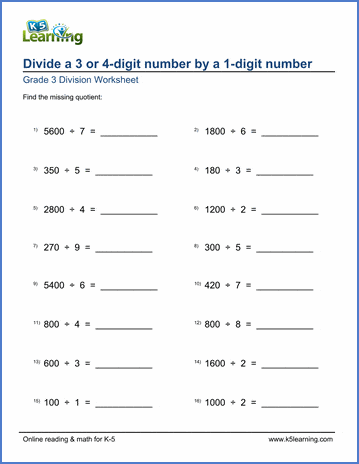# Divide 3 or 4-digit numbers by 1-digit numbers

## Math worksheets: divide a 3 or 4-digit number by a 1-digit number (no remainders)

Below are six versions of our grade 3 math worksheet on dividing a 3 or 4-digit number by a 1-digit number.  Answers do not have remainders and are either single digits, tens or hundreds.  Students should attempt to answer these questions "mentally" without writing down intermediary steps.  These worksheets are pdf files.## More division worksheets

Explore all of our division worksheets, from simple division facts to long division of large numbers.

## What is K5?

K5 Learning offers reading and math worksheets, workbooks and an online reading and math program for kids in kindergarten to grade 5.  We help your children build good study habits and excel in school.